# Creating Spreadsheets with OpenPyXL and Python

Reading Excel spreadsheets is all well and good. However, you also need to be able to create or edit a spreadsheet. The focus of this chapter will be on learning how to do that! OpenPyXL lets you create Microsoft Excel spreadsheets with a minimum of fuss.

Creating Excel spreadsheets using Python allows you to generate a new type of report that your users will use. For example, you might receive your data from a client in the form of JSON or XML. These data formats are not something that most accountants or business people are used to reading.

Once you learn how to create Excel spreadsheets with Python, you can leverage that knowledge to transform other data into Excel spreadsheets. This knowledge also allows you to do the reverse, taking in an Excel spreadsheet and output a different format, such as JSON or XML.

In this article, you will learn how to use OpenPyXL to do the following:

• Insert and delete rows and columns
• Edit cell data
• Create merged cells
• Fold rows and columns

Let’s get started by creating a brand new spreadsheet!

Creating an empty spreadsheet using OpenPyXL doesn’t take much code. Open up your Python editor and create a new file. Name it `creating_spreadsheet.py`.

```# creating_spreadsheet.py

from openpyxl import Workbook

def create_workbook(path):
workbook = Workbook()
workbook.save(path)

if __name__ == "__main__":
create_workbook("hello.xlsx")```

The critical piece here is that you need to import the `Workbook` class. This class allows you to instantiate a `workbook` object that you can then save. All this code does is create the file that you pass to it and save it.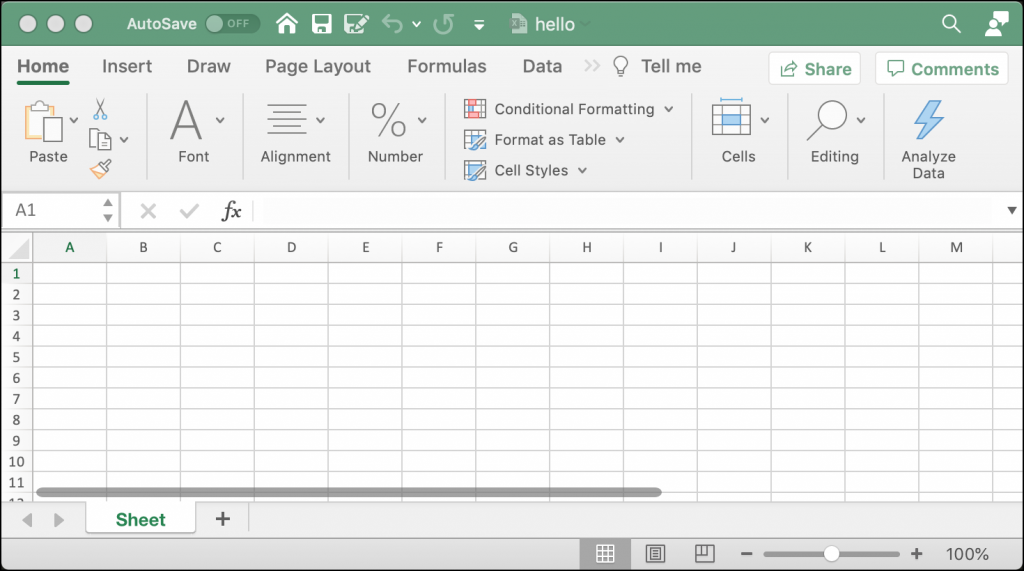When writing data in a spreadsheet, you need to get the “sheet” object. You learned how to do that in the previous chapter using `workbook.active`, which gives you the active or currently visible sheet. You could also explicitly tell OpenPyXL which sheet you want to access by passing it a sheet title.

For this example, you will create another new program and then use the active sheet. Open up a new file and name it `adding_data.py`. Now add this code to your file:

```# adding_data.py

from openpyxl import Workbook

def create_workbook(path):
workbook = Workbook()
sheet = workbook.active
sheet["A1"] = "Hello"
sheet["A2"] = "from"
sheet["A3"] = "OpenPyXL"
workbook.save(path)

if __name__ == "__main__":
create_workbook("hello.xlsx")```

This code will overwrite the previous example’s Excel spreadsheet. After you create the `Workbook()` object, you grab the active Worksheet. Then you add text strings to the cells: A1, A2, and A3. The last step is to save your new spreadsheet.

When you run this code, your new spreadsheet will look like this: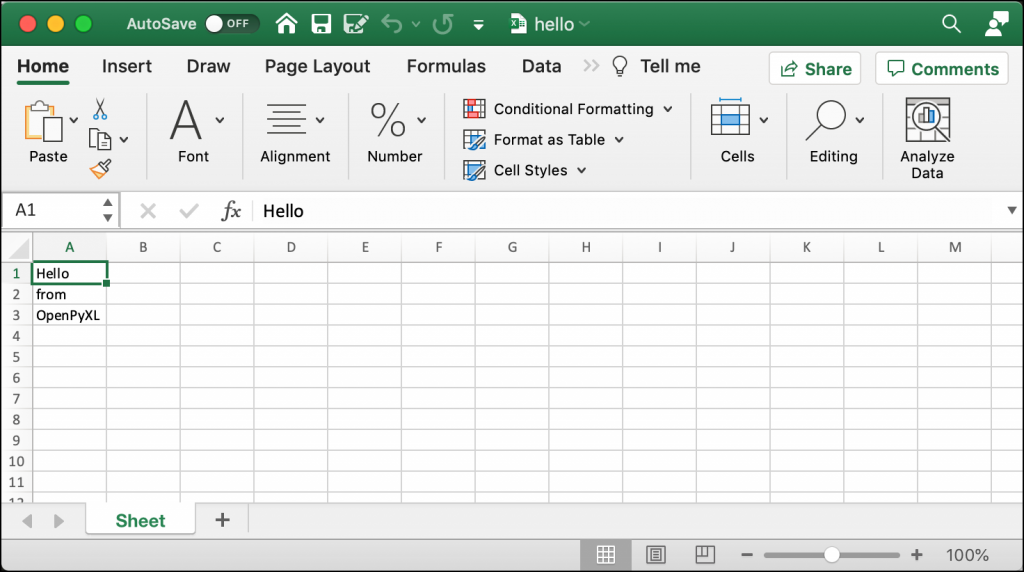You can use this technique to write data to any cell in your spreadsheet.

Now let’s find out how to add and remove a worksheet!

Adding a worksheet to a workbook happens automatically when you create a new Workbook. The Worksheet will be named “Sheet” by default. If you want, you can set the name of the sheet yourself.

To see how this works, create a new file named `creating_sheet_title.py` and add the following code:

```# creating_sheet_title.py

from openpyxl import Workbook

def create_sheets(path):
workbook = Workbook()
sheet = workbook.active
sheet.title = "Hello"
sheet2 = workbook.create_sheet(title="World")
workbook.save(path)

if __name__ == "__main__":
create_sheets("hello_sheets.xlsx")```

Here you create the `Workbook` and then grab the active Worksheet. You can then set the Worksheet’s title using the `title` attribute. The following line of code adds a new worksheet to the Workbook by calling `create_sheet()`.

The `create_sheet()` method takes two parameters: `title` and `index`. The `title` attribute gives a title to the Worksheet. The `index` tells the `Workbook` where to insert the Worksheet, from left to right. If you specify zero, your Worksheet gets inserted at the beginning.

If you run this code, your new spreadsheet will look like this: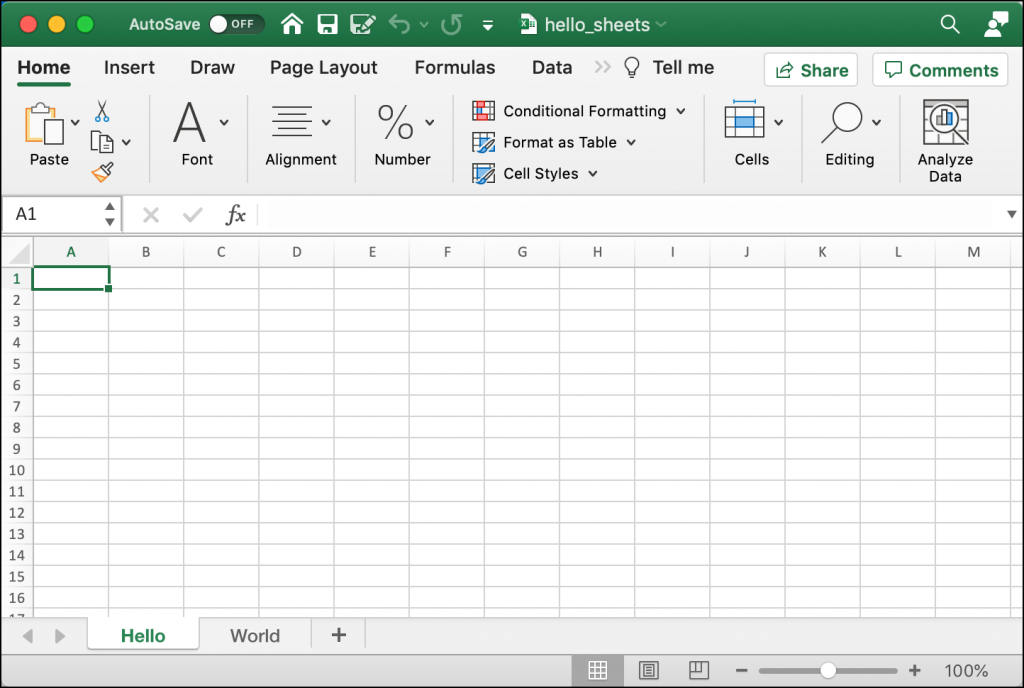Sometimes you will need to delete a worksheet. Perhaps that sheet no longer has valid information, or it was created by accident.

To see how to delete a worksheet, create another new file and name it `delete_sheets.py`. Then add this code:

```# delete_sheets.py

import openpyxl

def create_worksheets(path):
workbook = openpyxl.Workbook()
workbook.create_sheet()
print(workbook.sheetnames)
# Insert a worksheet
workbook.create_sheet(index=1, title="Second sheet")
print(workbook.sheetnames)
del workbook["Second sheet"]
print(workbook.sheetnames)
workbook.save(path)

if __name__ == "__main__":
create_worksheets("del_sheets.xlsx")```

In this example, you create two new sheets. The first Worksheet has no title specified, so it defaults to “Sheet1”. You supply a title to the second sheet, and then you print out all the current worksheet titles.

Next, you use Python’s `del` keyword to delete the Worksheet’s name from the workbook, which removes the sheet. Then you print out the current worksheet titles again.

Here is the output from running the code:

```['Sheet', 'Sheet1']
['Sheet', 'Second sheet', 'Sheet1']
['Sheet', 'Sheet1']```

The first Worksheet gets created automatically when you instantiate the `Workbook`. The Worksheet is named “Sheet”. Then you make “Sheet1”. Lastly, you create “Second sheet”, but you insert it at position 1, which tells the Workbook to shift ‘Sheet1’ to the right by one position.

You can see from the output above how the worksheets are ordered before and after you add and delete the “Second sheet”.

Now let’s learn about inserting and removing rows and columns!

## Inserting and Deleting Rows and Columns

The OpenPyXL package provides you with several methods that you can use to insert or delete rows and columns. These methods are a part of the `Worksheet` object.

You will learn about the following four methods:

• `.insert_rows()`
• `.delete_rows()`
• `.insert_cols()`
• `.delete_cols()`

Each of these methods can take these two arguments:

• `idx` – The index to insert into (or delete from)
• `amount` – The number of rows or columns to add (or delete)

You can use the insert methods to insert rows or columns at the specified index.

Open up a new file and name it `insert_demo.py`. Then enter this code in your new file:

```# insert_demo.py

from openpyxl import Workbook

def inserting_cols_rows(path):
workbook = Workbook()
sheet = workbook.active
sheet["A1"] = "Hello"
sheet["A2"] = "from"
sheet["A3"] = "OpenPyXL"
# insert a column before A
sheet.insert_cols(idx=1)
# insert 2 rows starting on the second row
sheet.insert_rows(idx=2, amount=2)
workbook.save(path)

if __name__ == "__main__":
inserting_cols_rows("inserting.xlsx")```

Here you create another new Spreadsheet. In this case, you add text to the first three cells in the “A” column. Then you insert one column at index one. That means you inserted a single column before “A”, which causes the cells in column “A” to shift to column “B”.

Next, you insert two new rows starting at index two. This code will insert two rows between the first and second rows.

You can see how this changes things by taking a look at the following screenshot: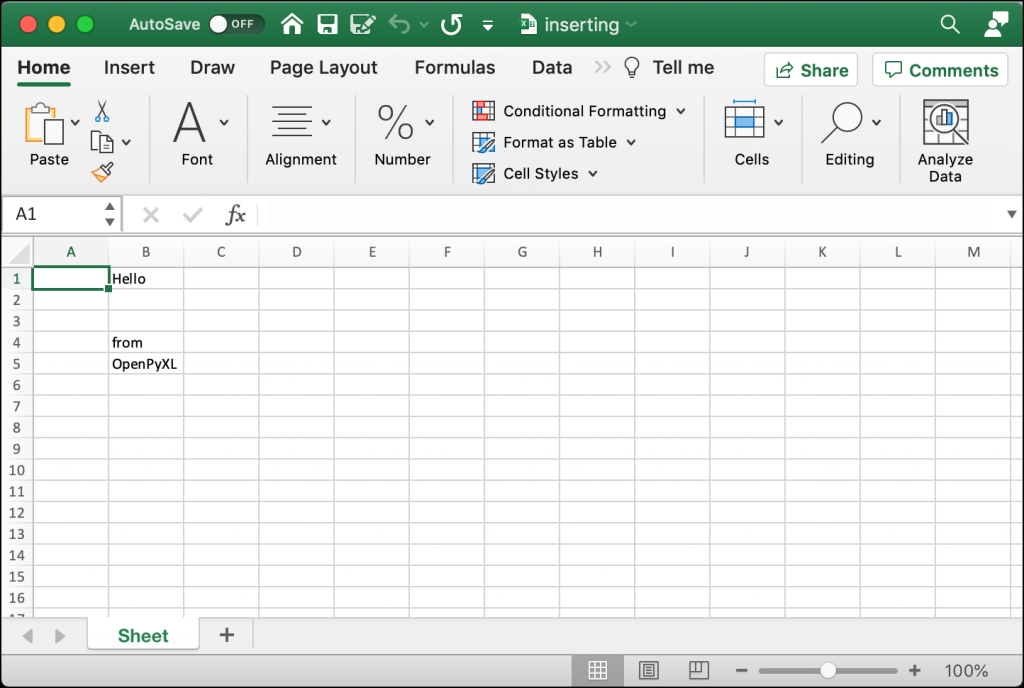Try changing the indexes or number of rows and columns that you want to insert and see how it works.

You will also need to delete columns and rows from time to time. To do that you will use `.delete_rows()` and `.delete_cols()`.

Open up a new file and name it `delete_demo.py`. Then add this code:

```# delete_demo.py

from openpyxl import Workbook

def deleting_cols_rows(path):
workbook = Workbook()
sheet = workbook.active
sheet["A1"] = "Hello"
sheet["B1"] = "from"
sheet["C1"] = "OpenPyXL"
sheet["A2"] = "row 2"
sheet["A3"] = "row 3"
sheet["A4"] = "row 4"
# Delete column A
sheet.delete_cols(idx=1)
# delete 2 rows starting on the second row
sheet.delete_rows(idx=2, amount=2)
workbook.save(path)

if __name__ == "__main__":
deleting_cols_rows("deleting.xlsx")```

In this example, you add text to six different cells. Four of those cells are in column “A”. Then you use `delete_cols()` to delete column “A”! That means you got rid of four values. Next, you delete two rows, starting at row number two.

When you run this code, your result should look like this: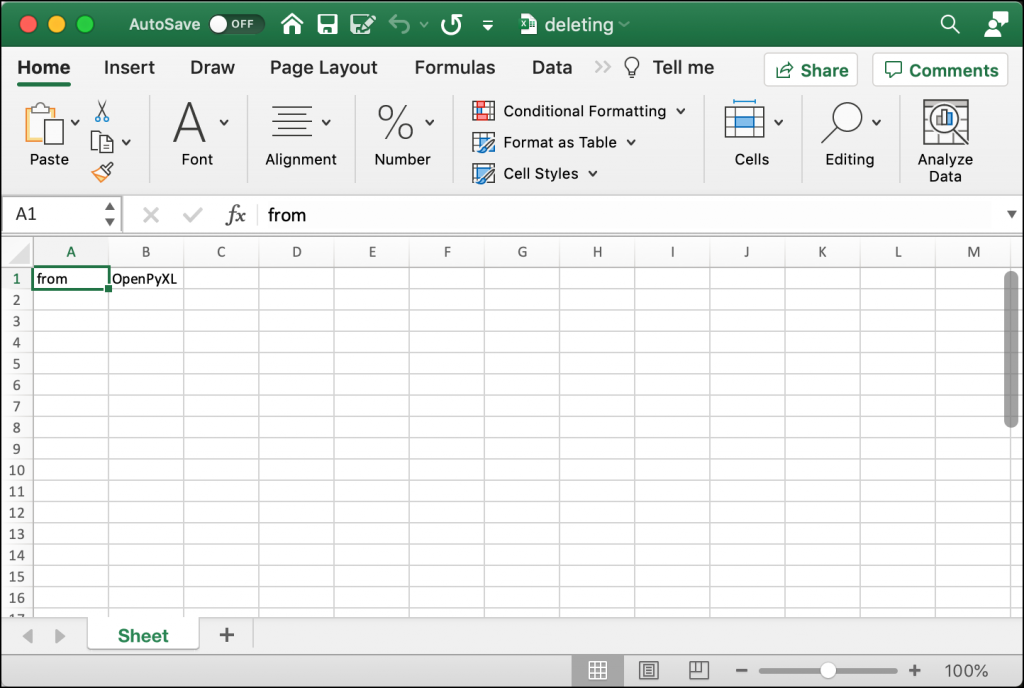Try editing the index or amount values to get familiar with deleting rows and columns.

## Editing Cell Data

You can use OpenPyXL to change the values in a pre-existing Excel spreadsheet. You can do that by specifying the cell you want to change and then setting it to a new value.

For this example, you will use the `inserting.xlsx` file you created in the previous section. Now create a new Python file named `editing_demo.py`. Then add the following code:

```# editing_demo.py

def edit(path, data):
sheet = workbook.active
for cell in data:
current_value = sheet[cell].value
sheet[cell] = data[cell]
print(f'Changing {cell} from {current_value} to {data[cell]}')
workbook.save(path)

if __name__ == "__main__":
data = {"B1": "Hi", "B5": "Python"}
edit("inserting.xlsx", data)```

This code loads up the Excel file that you created in the previous section. It then loops over each value in the `data` dictionary that you passed to the `edit()` function. You get the current value for the cell using a key in the dictionary. Then you change that cell’s value to match the value in the dictionary.

To make it more obvious what is going on, you print out the old and new values of the cell.

When you run this code, you will see the following output:

```Changing B1 from Hello to Hi
Changing B5 from OpenPyXL to Python```

Open up the new version of the `inserting.xlsx` file, and it should now look like this: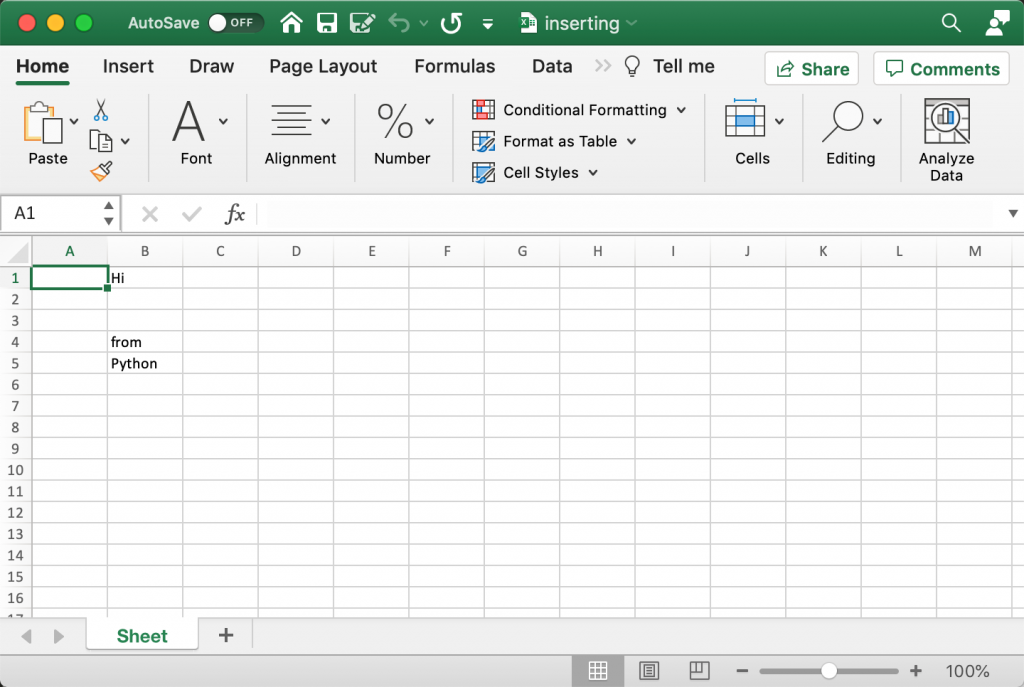Here you can see how the cell values have changed to match the one specified in the `data` dictionary.

Now you can move on and learn how to create merged cells!

## Creating Merged Cells

A merged cell is where two or more cells get merged into one. To set a MergedCell’s value, you have to use the top-left-most cell. For example, if you merge “A2:E2”, you would set the value of cell “A2” for the merged cells.

To see how this works in practice, create a file called `merged_cells.py` and add this code to it:

```# merged_cells.py

from openpyxl import Workbook
from openpyxl.styles import Alignment

def create_merged_cells(path, value):
workbook = Workbook()
sheet = workbook.active
sheet.merge_cells("A2:E2")
top_left_cell = sheet["A2"]
top_left_cell.alignment = Alignment(horizontal="center",
vertical="center")
sheet["A2"] = value
workbook.save(path)

if __name__ == "__main__":
create_merged_cells("merged.xlsx", "Hello World")```

OpenPyXL has many ways to style cells. In this example, you import `Alignment` from `openpyxl.styles`. You will learn more about styles and formatting cells in a later chapter.

Here you merge the cells “A2:E2” and set the alignment to the center of the cell. Then you set the value of “A2” to a string that you passed to the `create_merged_cells()` function.

When you run this example, your new Excel spreadsheet will look like this: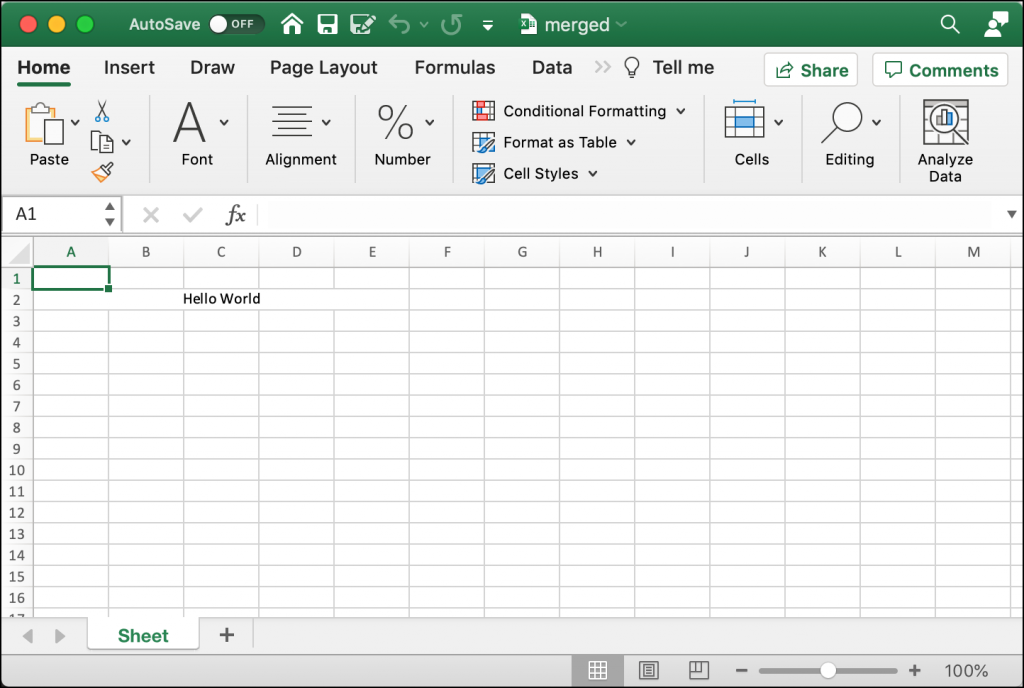To get some hands-on experience, change the range of cells you want to merge and try it with and without the alignment set.

## Folding Rows and Columns

Microsoft Excel supports the folding of rows and columns. The term “folding” is also called “hiding” or creating an “outline”. The rows or columns that get folded can be unfolded (or expanded) to make them visible again. You can use this functionality to make a spreadsheet briefer. For example, you might want to only show the sub-totals or the results of equations rather than all of the data at once.

OpenPyXL supports folding too. To see how this works, create a new file named `folding.py` and enter the following code:

```# folding.py

import openpyxl

def folding(path, rows=None, cols=None, hidden=True):
workbook = openpyxl.Workbook()
sheet = workbook.active

if rows:
begin_row, end_row = rows
sheet.row_dimensions.group(begin_row, end_row, hidden=hidden)

if cols:
begin_col, end_col = cols
sheet.column_dimensions.group(begin_col, end_col, hidden=hidden)

workbook.save(path)

if __name__ == "__main__":
folding("folded.xlsx", rows=(1, 5), cols=("C", "F"))```

Your `folding()` function accepts a tuple of rows or columns or both. You can tell OpenPyXL whether or not you want those rows and columns to be hidden, or folded. In this example, you fold rows 1-5 and columns C-F. To cause the folding to occur, you need to call `sheet.row_dimensions.group()`.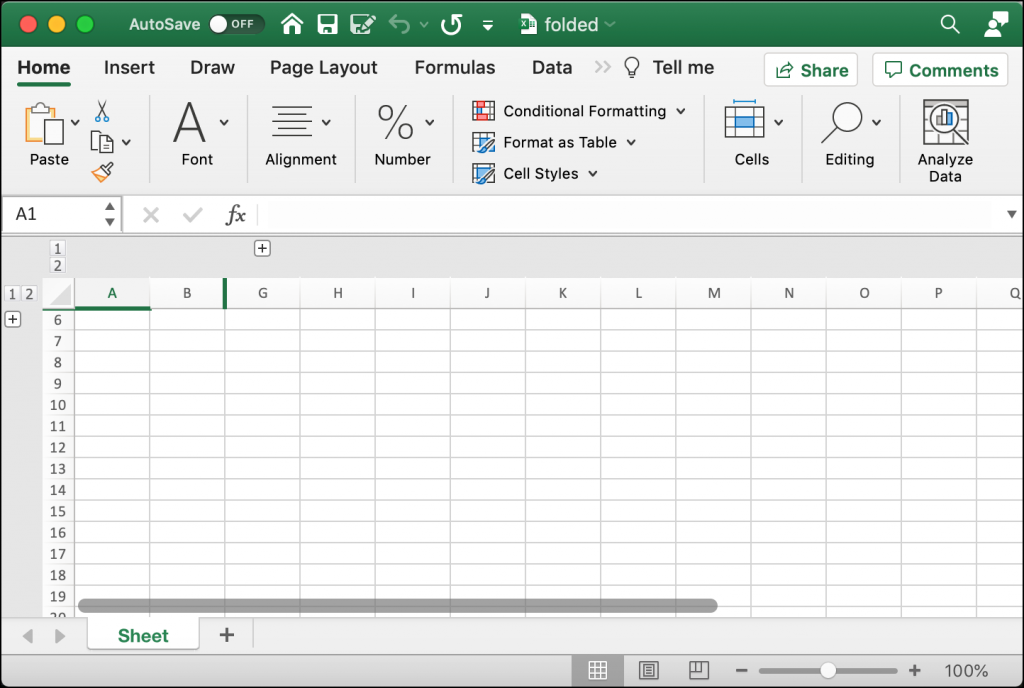You can see in this spreadsheet that some of the rows and columns are folded or hidden. There is a “+” symbol next to row 6 and another “+” symbol above column “G”. If you click on either of those buttons, it will expand the folded rows or columns.

Give this code a try. You can also experiment with different row or column ranges.

Now you are ready to learn how to freeze a pane!

## Freezing Panes

Microsoft Excel allows you to freeze panes. What that means is that you can freeze one or more columns or rows. One popular use case is to freeze a row of headers so that the headers are always visible while scrolling through a lot of data.

OpenPyXL provides a `freeze_panes` attribute on the Worksheet object that you can set. You need to select a cell below and to the right of the columns that you want to freeze. For example, if you want to freeze the first row in your spreadsheet, then you would select cell at “A2” to apply the freeze to that row.

You can see how this works by writing some code. Open up a new file and name it `freezing_panes.py`. Then enter the following into it:

```# freezing_panes.py

from openpyxl import Workbook

def freeze(path, row_to_freeze):
workbook = Workbook()
sheet = workbook.active
sheet.title = "Freeze"
sheet.freeze_panes = row_to_freeze
data = [dict(zip(headers, ("Mike", "123 Storm Dr", "IA", "50000"))),
row = 2
for d in data:
sheet[f'A{row}'] = d["Name"]
sheet[f'C{row}'] = d["State"]
sheet[f'D{row}'] = d["Zip"]
row += 1
workbook.save(path)

if __name__ == "__main__":
freeze("freeze.xlsx", row_to_freeze="A2")```

Here you create a new `Workbook` and set the active sheet’s title to “Freeze”. Then you set the `freeze_panes` attribute to “A2”. The rest of the code in the function adds a couple of rows of data to the Worksheet.

When you run this code, the spreadsheet that you create will look like this: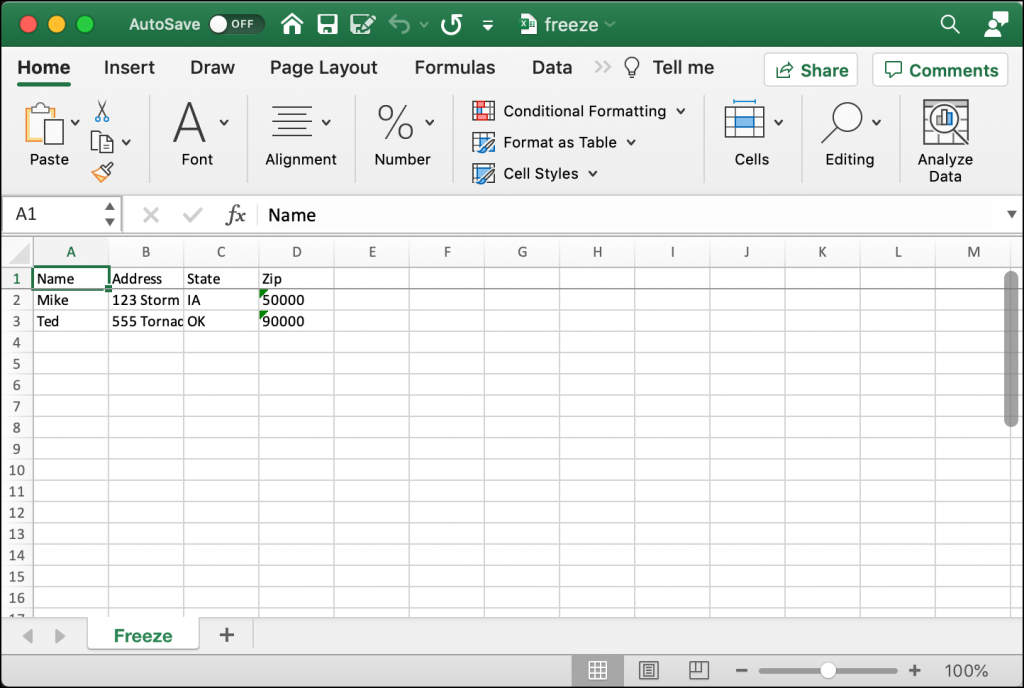Try scrolling down through some rows in the spreadsheet. The top row should always remain visible because it has been “frozen”.

## Wrapping Up

You can use OpenPyXL not only to create an Excel spreadsheet, but modify a pre-existing one. In this chapter, you learned how to do the following: